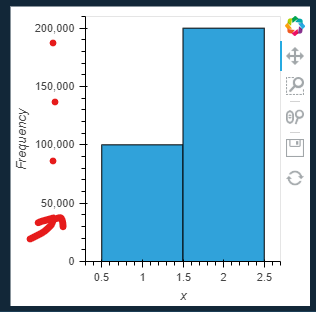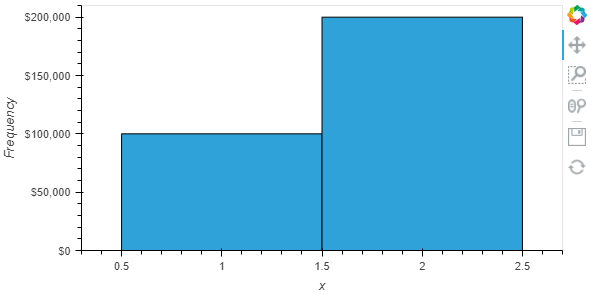# How do I format yaxis tick labels with comma as thousands seperator?

I would like to format my yaxis tick labels with comma as thousands seperator.

The below gets close

``````import holoviews as hv
hv.extension('bokeh')
data2 = {
"x": [1,2],
"Frequency": [100000, 200000],
}
hv.Histogram(data2).opts(yformatter='%.0f')
``````

but does not add the commas.

If I change to `yformatter='%,.0f'` the plot just does not show.
`
It’s the same if I provide the function below to yformatter

``````def format_number(x):
try:
return '{:,.0f}'.format(x)
except:
return '%d%' % x
``````

Any suggestions?

Problem solved using `NumeralTickFormatter(format="0,0")`.

``````import holoviews as hv
hv.extension('bokeh')
from bokeh.models.formatters import NumeralTickFormatter
formatter = NumeralTickFormatter(format="0,0")
data2 = {
"x": [1,2],
"Frequency": [100000, 200000],
}
hv.Histogram(data2).opts(yformatter=formatter)
``````1 Like

What if I want to add \$?

`df_with_salaries.hvplot.kde(y='average_salary', xformatter=formatter)`

Add the \$ in the format string:

``````formatter = NumeralTickFormatter(format="\$0,0")

``````1 Like

of course. Thanks a lot.

1 Like